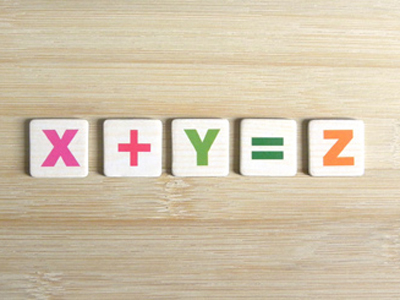In these questions, numbers are represented by letters.

# VR - Working Out Sums Using Letters

Working Out Sums Using Letters may sound frightening to some! Is it some fiendish code requiring a genius’ mind to solve? Well no, it is in fact quite a simple idea.

The questions are just basic maths, but with a difference: numerical values are given to letters. The answers to these questions must also be assigned a letter.

## How Are These Questions Posed In The Exam?

Candidates are told that certain letters have the same value as named numbers. They are then shown a calculation made up of letters rather than numbers, and asked to fill in the answer.

This explanation makes it sound quite complicated, but it really isn’t. The best way to show you is with some examples.

Example Question One

What is the answer to the sum, written as a letter?

If A = 7, B = 8, C = 18, D = 2 and E = 9

C ÷ D - A =

All you have to do is write this out – or do it mentally – using the figures given and then transfer the answer back into a letter.

Using the code we are given, the sum looks like this:

18 ÷ 2 - 7 = 2

The code letter for 2 is D, so that is the correct answer.

## Technique Tip:

Some of you may remember an acronym from GCSE/O Level maths which showed you which order to carry out operations in mathematical questions (BIDMAS or BODMAS). It said that you should carry out the division before the multiplication and then do the addition etc. Forget that for these questions. Carry out each sum starting at the beginning and work your way through unless told otherwise.

Example Question Two

What is the answer to the sum, written as a letter?

If A = 17, B = 8, C = 10, D = 22 and E = 6

A x E - D ÷ C =

As the technique tip states, start at the beginning and work your way through the sum. The first part is the hardest and it would help your child to write down the answer unless they are a maths whizz. If they need to write it out as a sum that’s normal, but if they are foxed by a sum of this kind they are going to really struggle in the maths paper.

17 x 6 - 22 ÷ 10 = 8

(17 x 6 = 102
102 - 22 = 80
80 ÷ 10 = 8)

‘B’ is the letter worth 8, so that is the answer.

If your child is practising this sort of question, it's easy to change the sum to make a new one and get them to find a different answer but with the same set of figures. If you're comfortable with numbers you can write your own letter sums faster than your child can answer them - nothing like a bit of variation!

## Sample Tests

At Education Quizzes we have four quizzes on working out sums using letters. Use them to practise if necessary, before the exam.

You’ll find them in the Eleven Plus Verbal Reasoning section of our site or, alternatively, you can follow these links:

Letters for Numbers 1

Letters for Numbers 2

Letters for Numbers 3

Letters for Numbers 4

Once your child has the general idea of how these questions work, then they should not pose a problem. Anybody with the strength in maths expected of a ten-year-old should be able to do these. If your child is struggling, then perhaps they would benefit from some of our Eleven Plus maths quizzes which you can find here:

Eleven Plus Maths

Good luck!

We use cookies to make your experience of our website better.

To comply with the new e-Privacy directive, we need to ask for your consent -